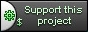# Applications Hierarchy

## General

The basic approach of mapping an URL to a member function of our class works well for small applications, but can become problematic for complex systems.

CppCMS allows you to create a hierarchy of applications that work as a single unit. You connect them by using the `url_mapper` and `url_dispatcher` classes.

## Example

### Hierarchy

We will create a very simple example of an "educational" application, that consists of two sub-applications:

• myapp - main application
• numbers - display different numbers:
• all
• odd
• even
• prime
• letters - display different letters:
• all
• capital
• small

We will create 3 classes where `myapp` would be the topmost class in the hierarchy, and `numbers` and `letters` would be sub-applications.

### Sub-Applications

Let's define our sub-applications:

```class numbers : public cppcms::application {
public:
```

The constructor:

```numbers(cppcms::service &srv) : cppcms::application(srv)
{
dispatcher().assign("",&numbers::all,this);
mapper().assign("");

dispatcher().assign("/odd",&numbers::odd,this);
mapper().assign("odd","/odd");

dispatcher().assign("/even",&numbers::even,this);
mapper().assign("even","/even");

dispatcher().assign("/prime",&numbers::prime,this);
mapper().assign("prime","/prime");
}
```

Create default application URL - all, and connect 4 member functions `odd`, `even`, `prime` and `all` to it, such that:

```void prime()
{
response().out() << "2,3,5,7,...";
}
void odd()
{
response().out() << "1,3,5,7,9,...";
}
void even()
{
response().out() << "2,4,6,8,10,...";
}
```

Now let's create our `all` member function that would include links to different modules:

```void all()
{
response().out()
<< "<a href='" << url("/")       << "'>Top</a><br>"
<< "<a href='" << url("/letters")<< "'>Letters</a><br>"
<< "<a href='" << url(".")       << "'>All Numbers</a><br>"
<< "<a href='" << url("odd")     << "'>Odd Numbers</a><br>"
<< "<a href='" << url("even")    << "'>Even Numbers</a><br>"
<< "<a href='" << url("prime")   << "'>Prime Numbers</a><br>"
<< "1,2,3,4,5,6,7,8,9,10,...";
}
```

Note:

• `url("/")` - points to the default location of the topmost application. The `numbers` application does not need to know what that is.
• `url("/letters")` - points to the default location of the sub-application letters starting from the root.
• `url(".")` - points to the default handling for the current application.
• `url("odd")`, `url("even")`, `url("prime")` - point to current application's different parts.

Now we create a similar class for `letters` class letters : public cppcms::application { public:

Constructor:

```letters(cppcms::service &srv) : cppcms::application(srv)
{
dispatcher().assign("",&letters::all,this);
mapper().assign("");

dispatcher().assign("/capital",&letters::capital,this);
mapper().assign("capital","/capital");

dispatcher().assign("/small",&letters::small,this);
mapper().assign("small","/small");

}
```

Member functions:

```void all()
{
response().out()
<< "<a href='" << url("/")       << "'>Top</a><br>"
<< "<a href='" << url("/numbers")<< "'>Numbers</a><br>"
<< "<a href='" << url(".")       << "'>All Letters</a><br>"
<< "<a href='" << url("capital") << "'>Capital Letters</a><br>"
<< "<a href='" << url("small")   << "'>Small Letters</a><br>"
<< "Aa, Bb, Cc, Dd,...";
}

void capital()
{
response().out() << "A,B,C,D,...";
}
void small()
{
response().out() << "a,b,c,d,...";
}
```

### Main Application

Now lets create our topmost application:

```class myapp: public cppcms::application {
public:
```

Constructor:

```myapp(cppcms::service &srv) :
cppcms::application(srv)
{
attach( new numbers(srv),
"numbers", "/numbers{1}", // mapping
"/numbers(/(.*))?", 1);   // dispatching
attach( new letters(srv),
"letters", "/letters{1}", // mapping
"/letters(/(.*))?", 1);   // dispatching

dispatcher().assign("",&myapp::describe,this);
mapper().assign(""); // default URL

mapper().root("/myapp");
}
```

Note: we call `attach` member function with following parameters:

```new numbers(srv)
```

New sub-application - we pass the ownership to the parent so we don't need to handle its lifetime on our own.

```"numbers", "/numbers{1}"
```

Mapping - application named "numbers" is mapped according the the pattern "/numbers{1}" - such that each sub application's URL would replace the `{1}` placeholder.

```"/numbers(/(.*))?", 1)
```

The regular expression pattern for matching the sub-application. Note, unlike with member functions, exactly one sub-expression is expected - this captured subexpression is passed to the child's main function for further dispatching.

The same is done for the `letters` and `myapp` member functions.

Now lets analyze how an HTTP request `GET /myapp/numbers/prime` is parsed.

1. The web server splits `/myapp/numbers/prime` to `/myapp` as `SCRIPT_NAME` and `/numbers/prime` as `PATH_INFO`
2. `myapp` matches `/numbers/prime` against the `/numbers(/(.*))?` expression, and extracts `/prime` as the subexpression that is passed to `numbers`'s main function.
3. `/prime` is matched against `/prime` expression and `numbers::prime` function is called.

Now lets show the final touch - the `describe` function of `myapp`:

```void describe()
{
response().out()
<< "<a href='" << url("/numbers")<< "'>Numbers</a><br>"
<< "<a href='" << url("/letters")<< "'>Letters</a><br>"
<< "<a href='" << url("/numbers/odd")<< "'>Odd Numbers</a><br>";
}
```

URL("/numbers") - relates to the default URL of the "numbers" sub-application, and similar for `letters`. The URL("/numbers/odd`) refers to "odd" mapping of the "numbers" sub-application. Let's see how the mapping is done in detail.

1. A path `/numbers/odd` is split into "numbers" and "odd" tokens.
2. We search for "numbers" sub-application and find it.
3. In `numbers`'s sub-application mapper we search for "odd" mapping and find it.
4. We construct all backward:

• "odd" -> "/odd"
• "/numbers{1}" + "/odd" -> "/numbers/odd"
• Root "/myapp" + "/numbers/odd" -> "/myapp/numbers/odd"

So we fully connect our main applications and sub-applications using some generic names.

## Running

Finally let's add the main function

```int main(int argc,char ** argv)
{
try {
cppcms::service app(argc,argv);
app.applications_pool().mount(cppcms::applications_factory<myapp>());
app.run();
}
catch(std::exception const &e) {
std::cerr<<e.what()<<std::endl;
}
}
```

Create the configuration file:

```{
"service" : {
"api" : "http",
"port" : 8080
},
"http" : {
"script" : "/myapp"
}
}
```

Build and run as:

```./myapp -c config.js
```

And hit the URL: http://localhost:8080/myapp

CppCMS is a web development framework for performance demanding applications.

## Support This Project